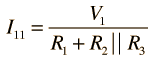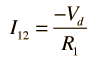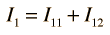# Superposition: Two Loop Problem

To apply the superposition theorem to calculate the current through resistor R1 in the two loop circuit shown, the individual current supplied by each battery is calculated with the other battery replaced by a short circuit.For R1 =Ω, R2 = Ω, R3 = Ω,

 and voltages V1 = V and V2 = V,

the calculated currents are= A,= A
 with a resultant current in R1 of= A.

Note: To avoid dealing with so many short circuits, any resistor with value zero will default to 1 when a voltage is changed. It can be changed back to a zero value if you wish to explore the effects of short circuits. Ohms and amperes are the default units, but if you put in resistor values in kilohms, then the currents will be milliamperes.

 Other approaches to two-loop circuits
Index

DC Circuits

 HyperPhysics***** Electricity and Magnetism R Nave
Go Back

# Superposition Theorem

The total current in any part of a linear circuit equals the algebraic sum of the currents produced by each source separately.

To evaluate the separate currents to be combined, replace all other voltage sources by short circuits and all other current sources by open circuits.

 Application to two-loop problem
Index

DC Circuits

 HyperPhysics***** Electricity and Magnetism R Nave
Go Back